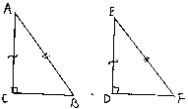# Chapter 10 Congruent Triangles RD Sharma Solutions Exercise 10.5 Class 9 Maths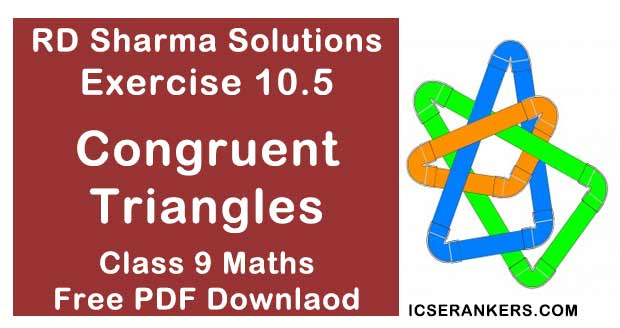Chapter Name RD Sharma Chapter 10 Congruent Triangles Exercise 10.5 Book Name RD Sharma Mathematics for Class 10 Other Exercises Exercise 10.1Exercise 10.2Exercise 10.3Exercise 10.4Exercise 10.6 Related Study NCERT Solutions for Class 10 Maths

### Exercise 10.5 Solutions

1. ABC is a triangle and D is the mid - point of BC. The perpendiculars from D to AB and AC are equal. Prove that the triangle is isosceles.

Solution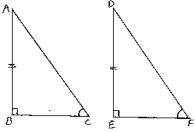Given that, in two right triangles one side and acute angle of one are equal to the corresponding side and angle of the other
We have to prove that the triangles are congruent
Let us consider two right triangles such that
∠B = ∠E = 90° ...(1)
AB = DE  ...(2)
∠C = ∠F  ...(3)
Now observe the two triangles ABC and DEF
∠C = ∠F   [From (3)]
∠B = ∠E   [From (1)]
and AB = DE  [From (2)]
So, by AAS congruence criterion, we have
ΔABC ≅ ΔDEF
∴ The two triangles are congruent
Hence, proved.

2. ABC is a triangle in which BE and CF are, respectively, the perpendiculars to the sides AC and AB. If BE = CF, prove that ΔABC is isosceles.
Solution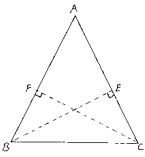Given that ABC is a triangle in which BE and CF are perpendicular to the sides AC and AB respectively such that BE = CF.
We have to prove that ΔABC is isosceles
Now, consider ΔBCF and ΔCBE,
We have
∠BFC = ∠CEB = 90°  [Given]
BC = CB   [Common side]
And CF = BE  [Given]
So, by RHS congruence criterion, we have  ΔBFC ≅ ΔCEB
Now,
∠FBC = ∠EBC   [∵ Incongruent triangles corresponding parts are equal]
⇒ ∠ABC = ∠ACB
⇒ AC = AB   [∵ Opposite sides to equal angles are equal in a triangle]
∴ ΔABC is isosceles

3. If perpendiculars from any point within an angle on its arms are congruent, prove that it lies on the bisector of that angle.
Solution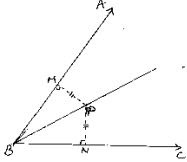Given that, if perpendicular from any point within, an angle on its arms is congruent, prove that it lies on the bisector of that angle
Now,
Let us consider an angle ABC and let BP be one of the arm within the angle
Draw perpendicular PN and PM on the arms BC and BA such that they meet BC and BA in N and M respectively.
Now, in ΔBPM and ΔBPN
We have ∠BMP = BNP = 90°  [given]
BP = BP  [Common side]
And MP = NP  [given]
So, by RHS congruence criterion, we have
ΔBPM ≅ ΔBPN
Now,
∠MBP = ∠NBP   [∵ Corresponding parts of congruent triangles are equal]
⇒ BP is the angular bisector of ∠ABC.
∴ Hence, proved.

4. In fig. 10.99, AD ⊥ CD and CB ⊥ CD . If AQ = BP and DP = CQ, prove that ∠DAQ = ∠CBP.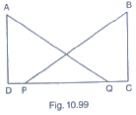SolutionGiven that, in the figure AD ⊥ CD and CB ⊥ CD and AQ = BP, DP = CQ
We have to prove that ∠DAQ = ∠CBP
Given that DP = QC
Given that DP = QC
⇒ DP + PQ = PQ + QC
⇒ DQ = PC ...(1)
Now, consider triangle DAQ and CBP,
We have
∠ADQ = ∠BCP = 90°  [given]
AQ = BP   [given]
And DQ = PC  [From (1)]
So, by RHS congruence criterion, we have ΔADQ ≅ ΔCBP
Now,
∠DAQ = ∠CBP  [∵ Corresponding parts of congruent triangles are equal]
∴ Hence proved

5. ABCD is a square, X and Y are points on sides AD and BC respectively such that AY = BX. Prove that BY = AX and ∠BAY = ∠4BX.
Solution
Given that ABCD is a square, X and Y are points on sides AD and BC respectively such that
AY = BX.
We have to prove BY  = AX and ∠BAY = ∠ABX
Join B and X, A and Y.
Since, ABCD is a square
⇒ ∠DAB  = ∠CBA = 90°
⇒ ∠XAB = ∠YBA = 90°  ...(1)
Now, consider triangle XAB and YBA
We have
∠XAB = ∠YBA = 90°  [From (1)]
BX = AY  [given]
And AB = BA  [Common side]
So, by RHS congruence criterion, we have ΔXAB ≅ ΔYBA
Now, we know that corresponding parts of congruent triangles are equal.
∴ BY = AX and ∠BAY = ∠ABX
∴ Hence proved

6. Which of the following statements are true (T) and which are false(F) :
(i) Sides opposite to equal angles of a triangle may be unequal.
(ii) Angles opposite to equal sides of a triangle are equal
(iii) The measure of each angle of an equilateral triangle is 60°
(iv) If the altitude from one vertex of a triangle bisects the opposite side, then the triangle may be isosceles.
(v) The bisectors of two equal angles of triangle are equal.
(vi) If the bisector of the vertical angle of a triangle bisects the base, then the triangle may be isosceles.
(vii) The two altitude corresponding to two equal sides of a triangle need not be equal.
(viii) If any two sides of a right triangle are respectively equal to two sides of other right triangle, then the two triangles are congruent.
(ix) Two right triangles are congruent if hypotenuse and a side of one triangle are respectively equal to the hypotenuse and a side of the other triangle.
Solution
(i) False (F)
Reason: Sides opposite to equal angles of a triangle are equal

(ii) True (F)
Reason : Since the sides are equal, the corresponding opposite angles must be equal

(iii) True(T)
Reason : Since all the three angles of an equilateral triangles are equal and sum of the three angles is 180°, each angle will be equal to 180°/3 = 60°

(iv) False (F)
Reason : Here the altitude from three vertex is also the perpendicular bisector of the opposite side.
The triangle must be isosceles and may be an euilateral triangle.

(v) True (T)
Reason : Since it an isosceles triangle, the lengths of bisectors of the two equal angles are equal

(vi) False (F)
Reason : The angular bisector of the vertex angle is also a median
⇒ The triangle must be an isosceles and also may be an euilateral triangle.

(vii) False (F)
Reason : Since two sides are equal, the triangle is an isosceles triangle.
The two altitudes corresponding to two equal sides must be equal.

(viii) False (F)
Reason : The two right triangles may or may not be congruent

(ix) True (T)
Reason : According to RHS congruence criterion the given statement is true.

7. Fill the blanks in the following so that each of the following statements is true.
(i) Sides opposite to equal angles of a triangle are ......
(ii) Angle opposite to equal sides of a triangle are .....
(iii) In an equilateral triangle all angles are .....
(iv) In a ΔABC if ∠A = ∠C, then AB = .........
(v) If altitudes CE and BF of a triangle ABC are equal, then AB =  ........
(vi) In an isosceles triangle ABC with AB = AC, if BD and CE are its altitudes, then BD is ........ CE.
(vii) In right triangles ABC and DEF, if hypotenuse AB = EF and side AC = DE, then ΔABC ≅ Δ .........
Solution
(i) Sides opposite to equal angles of a triangle are equal.

(ii) Angles opposite to equal sides of a triangle are equal.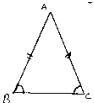(iii) In an equilateral triangle all angles are equal
Reason: Since all sides are equal in a equilateral triangle, the angles opposite to equal sides will be equal.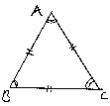(iv) In a ΔABC if ∠A = ∠C, then AB = BC
Reason : Since, the sides opposite to equal angles are equal, the side opposite to ∠A i.e., BC and ∠C i.e., AB are equal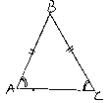(v) If altitudes CE and BF of a triangle ABC are equal, then
AB = AC
Reason : From RHS congruence criterion ΔBEC ≅ ΔCFB
⇒ ∠EBC = ∠FCB ⇒ ∠ABC = ∠ACB ⇒ AC = AB
[∵ Sides opposite to equal angels are equal]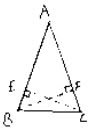(vi) In an isosceles triangle ABC with AB = AC, if BD and CE are its altitudes, then BD is equal to CE
Reason: Since angles opposite to equal sides are equal, so
∠ABC = ∠ACB
⇒∠EBC = ∠DCB
So, by ASA congruence criterion
ΔEBC ≅ ΔDCB
⇒ CE = BD  [Corresponding parts of congruent triangles are equal]

(vii) In right triangles ABC and DEF, if hypotenuse AB = EF and side AC = DE, then.
ΔABC ≅  ΔEFD
Reason: From RHS congruence criterion we have ΔABC ≅ ΔEFD